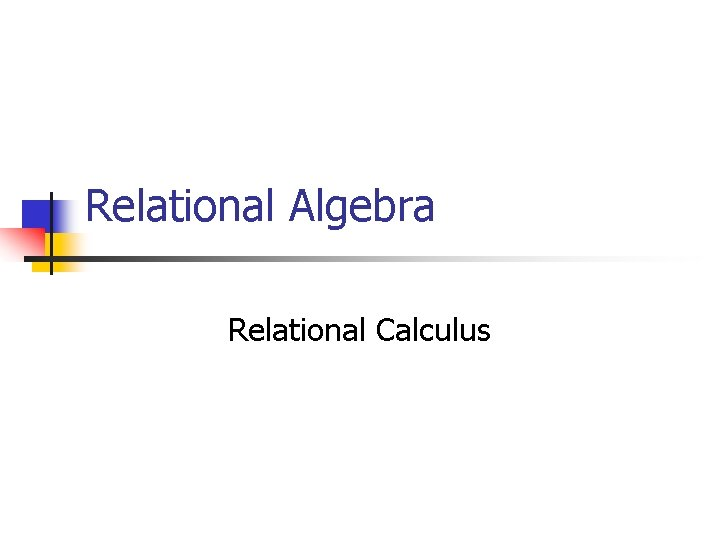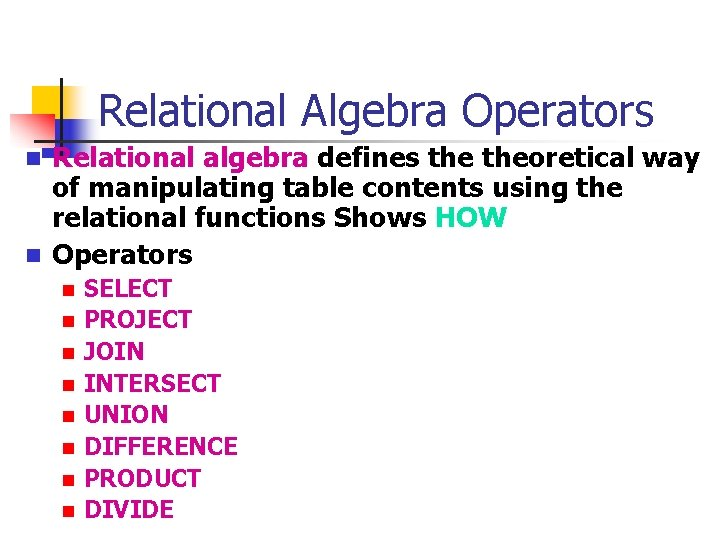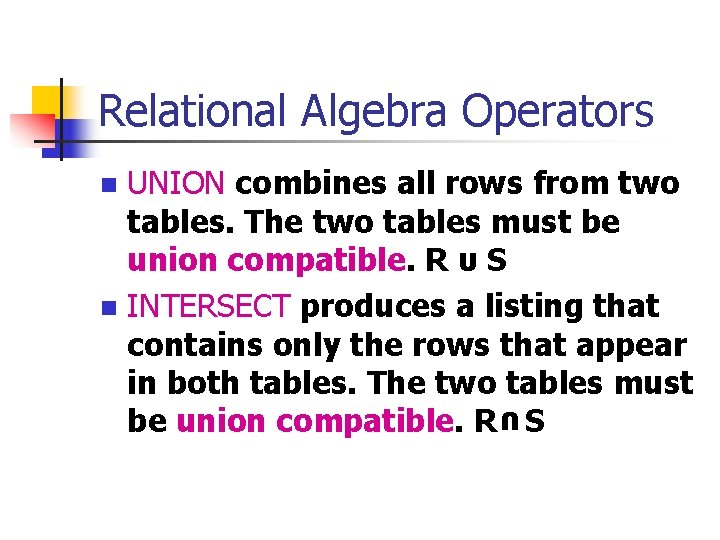# Relational Algebra Relational Calculus Relational Algebra Operators n

• Slides: 9
Download presentationRelational Algebra Relational CalculusRelational Algebra Operators n n Relational algebra defines theoretical way of manipulating table contents using the relational functions Shows HOW Operators n n n n SELECT PROJECT JOIN INTERSECT UNION DIFFERENCE PRODUCT DIVIDERelational Algebra Operators n SELECT yields values for all attributes found in a table. It yields a horizontal subset of a table. σpredicate (R) n PROJECT produces a list of all values for selected attributes. It yields a vertical subset of a table. Πa 1…an (R) n DIFFERENCE yields all rows in one table that are not found in the other table; i. e. , it subtracts one table from the other. The tables must be union compatible. R - SRelational Algebra Operators n n n A PRODUCT produces a list of all possible pairs of rows from two tables. Default is CARTESIAN PRODUCT R X S Constrain with: n n n A SELECT is performed to yield only the rows for which the common attribute values match. A PROJECT is performed to yield a single copy of each attribute, thereby eliminating duplicate column. DIVIDE requires the use of one singlecolumn table and one two-column table. R÷SRelational Algebra Operators UNION combines all rows from two tables. The two tables must be union compatible. R υ S n INTERSECT produces a listing that contains only the rows that appear in both tables. The two tables must be union compatible. R S n υRelational Algebra Operators JOIN allows us to combine information from two or more tables. JOIN is the real power behind the relational database, allowing the use of independent tables linked by common attributes. n Theta join R n Equijoin Function is = n Natural join One occurrence of common attribute eliminated. n Outer join R n Semijoin and project υ n FS S = σF(R X S) Usually leftRelational Database Software Classification Relational Database ClassificationRelational Calculus n n n Specifies WHAT is retrieved QBE Predicate calculus – truth value for each proposition n Tuple relational calculus Domain relational calculus {x|P(x)}Relational Calculus Well-formed formula –wff n n And, or, not Quantifiers n Existential quantifier n n E n Must be true for at least one instance Universal quantifier V n True for every instance